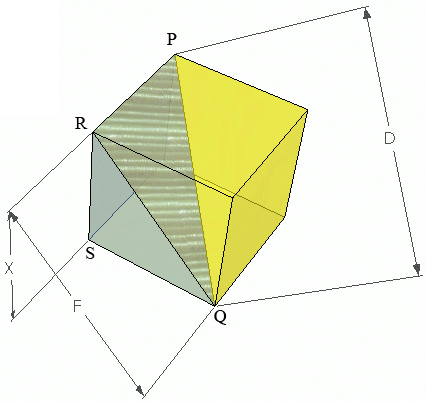SEARCH HOMEMath Central Quandaries & QueriesQuestion from Peter, a student: when u have a cube and the inner diagonal is 41.6 how long is the outside diagonalHi Peter,

In the diagram below $D$ s the length of an inner diagonal, $F$ is the length of an outside diagonal and $X$ is the length of a side.Triangles $QPR$ and $QRS$ are right triangles. Use the Pythagorean Theorem.

PennyMath Central is supported by the University of Regina and the Imperial Oil Foundation.